RRB JE EEE (CBT I) Mock Test- 6

# RRB JE EEE (CBT I) Mock Test- 6

Test Description

## 100 Questions MCQ Test RRB JE Mock Test Series Electrical & Electronics Engineering | RRB JE EEE (CBT I) Mock Test- 6

RRB JE EEE (CBT I) Mock Test- 6 for Railways 2023 is part of RRB JE Mock Test Series Electrical & Electronics Engineering preparation. The RRB JE EEE (CBT I) Mock Test- 6 questions and answers have been prepared according to the Railways exam syllabus.The RRB JE EEE (CBT I) Mock Test- 6 MCQs are made for Railways 2023 Exam. Find important definitions, questions, notes, meanings, examples, exercises, MCQs and online tests for RRB JE EEE (CBT I) Mock Test- 6 below.
Solutions of RRB JE EEE (CBT I) Mock Test- 6 questions in English are available as part of our RRB JE Mock Test Series Electrical & Electronics Engineering for Railways & RRB JE EEE (CBT I) Mock Test- 6 solutions in Hindi for RRB JE Mock Test Series Electrical & Electronics Engineering course. Download more important topics, notes, lectures and mock test series for Railways Exam by signing up for free. Attempt RRB JE EEE (CBT I) Mock Test- 6 | 100 questions in 90 minutes | Mock test for Railways preparation | Free important questions MCQ to study RRB JE Mock Test Series Electrical & Electronics Engineering for Railways Exam | Download free PDF with solutions
 1 Crore+ students have signed up on EduRev. Have you?
RRB JE EEE (CBT I) Mock Test- 6 - Question 1

### The ratio of the number of Matches played by Rohit and Kohli is 6:5. Kohli scored an average of 52 runs per match and Rohit's average is 25% less than that. If the total runs scored by Kohli is 546 more than Rohit, what is the number of matches Kohli has played?

Detailed Solution for RRB JE EEE (CBT I) Mock Test- 6 - Question 1 Let the number of matches played by Rohit and Kohli be 6k and 5k respectively.

Average runs scored in a match by Rohit = 52(1 - 25/100) = 39

Given, 52*5k - 39*6k = 546

=> 26k = 546

=> k = 21

Number of Matches played by Kohli = 5*21 = 105

RRB JE EEE (CBT I) Mock Test- 6 - Question 2

### What is the value of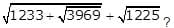Detailed Solution for RRB JE EEE (CBT I) Mock Test- 6 - Question 2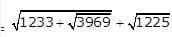= √(1233+63)+35

= 36 + 35

= 71

RRB JE EEE (CBT I) Mock Test- 6 - Question 3

### For what value of 'k', quadratic equation 4x2 - 3m√2x + k = 0 (where, m>0) has equal roots? (Given: 2m2 + 7m - 60 = 0)

Detailed Solution for RRB JE EEE (CBT I) Mock Test- 6 - Question 3 For 2m2 + 7m - 60 = 0

2m2 - 8m + 15m - 60 = 0

m = -15/2,

Quadratic equation 4x2 - 3m√2x + k = 0 has equal roots, therefore,

D = 0 = (-3m√2)2 - 4 x 4 x k

For m = 4:

18 x (4)2 = 16 x k

k = 18

RRB JE EEE (CBT I) Mock Test- 6 - Question 4

Study the following table carefully and answer the related question.

The following table represents the marks of the top four students of the class in four subjects.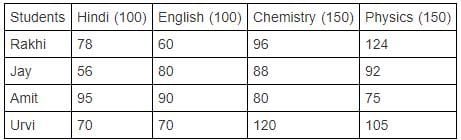Who got approximately 71.5% percentage in the class?

Detailed Solution for RRB JE EEE (CBT I) Mock Test- 6 - Question 4 Total marks = 100 + 100 + 150 + 150 = 500

Total marks of Rakhi = 78 + 60 + 96 + 124 = 358

Rakhi's percentage = (358/500) x 100 = 71.6%

Total marks of Jay = 56 + 80 + 88 + 92 = 316

Jay's percentage = (316/500) x 100 = 63.2%

Total marks of Amit = 95 + 90 + 80 + 75 = 340

Amit's percentage = (340/500) x 100 = 68%

Total marks of Urvi = 70 + 70 + 120 + 105 = 365

Urvi's percentage = (365/500) x 100 = 73%

RRB JE EEE (CBT I) Mock Test- 6 - Question 5

A sum of money at simple interest amounts to Rs 2240 in 2 years and to Rs 2600 in 5 years. What is the principal amount?

Detailed Solution for RRB JE EEE (CBT I) Mock Test- 6 - Question 5 Interest for 3 years = Rs 2600 - Rs 2240 = Rs 360

=> Interest for 2 years = 360x2/3 =Rs 240

Hence, principal = (2240 - 240) = Rs 2000

RRB JE EEE (CBT I) Mock Test- 6 - Question 6

Number of kids in 10 families is given below. Find the median of number of kids.

3, 4, 2, 1, 0, 2, 4, 0, 1 and 2

Detailed Solution for RRB JE EEE (CBT I) Mock Test- 6 - Question 6 Ascending order of number of kids in the family-

0, 0, 1, 1, 2, 2, 2, 3, 4, 4

Total terms = 10 = Even

Median = [(n/2)th term + {(n/2) + 1} th term]/2

=> [(10/2)th term + {(10/2) + 1}th term]/2

RRB JE EEE (CBT I) Mock Test- 6 - Question 7

There are four persons A, B,C and D, and the ratio of the present age of A to C is 4: 3, C is 7 years younger to E who is 16 years old at present. The present age of B is 75% of the present age of D and B is 6 years elder to A, what is the average of the present age of persons B, C and D?

Given below are the steps involved. Arrange them in the sequential order.

Let the age of A = 4A

(A) Age of A and C is 12 years and 9 years respectively.

(B) Present age of E = (3A + 7) = 16 => A = 3

(C) Required average = (18 + 9 + 24)/3 = 17 years

(D) Present age of B = 12 + 6 = 18 years and present age of D = 18 * (100/75) = 24 years

(E) Present age of C = 4A * (3/4) = 3A

Detailed Solution for RRB JE EEE (CBT I) Mock Test- 6 - Question 7 The correct order is

(E) Present age of C = 4A * (3/4) = 3A

(B) Present age of E = (3A + 7) = 16 => A = 3

(A) Age of A and C is 12 years and 9 years, respectively

(D) Present age of B = 12 + 6 = 18 years and present age of D = 18 * (100/75) = 24 years

(C) Required average = (18 + 9 + 24)/3 = 17 years

RRB JE EEE (CBT I) Mock Test- 6 - Question 8

The average age of a man and his son is 54 years. The ratio of their ages is 23:13. What will be the ratio of their ages after 6 years?

Detailed Solution for RRB JE EEE (CBT I) Mock Test- 6 - Question 8

Let their ages be 23x and 13x respectively.

The average age is 54 years which means the sum of their ages = 108 years.

i.e. 36x = 108

or x = 3

Hence their ages are 69 and 39 years, respectively.

After 6 years, their ages would be 75 and 45 years

So the required ratio = 75:45 = 5:3

RRB JE EEE (CBT I) Mock Test- 6 - Question 9

Out of 5 numbers, whose average is 36, the first one is one fifth of the sum of the last four. The first number is?

Detailed Solution for RRB JE EEE (CBT I) Mock Test- 6 - Question 9 Let the five numbers be a, b, c, d and e

Given, (a+b+c+d+e)/5=36

a+b+c+d+e = 180

a = (b+c+d+e)/5

a = (180-a)/5

a = 30

RRB JE EEE (CBT I) Mock Test- 6 - Question 10

What value we get when: √(92 - 43 + 3) is multiplied by √(25 * 10-1)?

Detailed Solution for RRB JE EEE (CBT I) Mock Test- 6 - Question 10

= √(92 - 43 + 3) * √(25 * 10-1)

= √(81 - 64 + 3) * √(32 ÷ 10)

= √20 * √3.

= √(20 * 3.2)

= √64

= 8

RRB JE EEE (CBT I) Mock Test- 6 - Question 11

Nidhi borrowed Rs 10000 from a bank for a period of two years at the rate of 10% per annum. From that amount, she invested Rs 3500 in another bank which offers simple interest @12.5% per annum and the remaining amount she invested into a chit fund which offers compound interest of 10% per annum. What is the profit obtained by Nidhi at the end of two years ?

Detailed Solution for RRB JE EEE (CBT I) Mock Test- 6 - Question 11 Total interest that Nidhi has to pay after 2 years =Rs (10000 X 2 X 10)/100 = Rs 2000

Interest obtained by Nidhi from the bank = Rs (3500 X 2 X 12.5)/100 = Rs 875

Interest obtained by Nidhi from Chit fund = 6500(1 + 0.1)2 - 6500 = Rs 1365

So total interest obtained by Nidhi = Rs (875 + 1365) = Rs 2240

Profit = Rs (2240 - 2000) = Rs 240

RRB JE EEE (CBT I) Mock Test- 6 - Question 12

If n is the greatest number that will divide the three numbers 1236, 3708 and 4944 leaving the same remainder in each case. What is the product of digits of 'n'?

Detailed Solution for RRB JE EEE (CBT I) Mock Test- 6 - Question 12 n = HCF of (3708 - 1236), (4944 - 3708) and (4944 - 1236)

= HCF of 2472, 1236 and 3708

= 1236

Product of digits of n = 1 x 2 x 3 x 6 = 36

RRB JE EEE (CBT I) Mock Test- 6 - Question 13

LCM of three numbers is 840 and their ratio is 2: 3: 5 respectively. What will be the HCF of these numbers?

Detailed Solution for RRB JE EEE (CBT I) Mock Test- 6 - Question 13 Let the three numbers be 2a, 3a and 5a respectively.

According to the question:

840 = 2a x 3a x 5a

a = 28

Therefore, the numbers are (2 x 28), (3 x 28) and (5 x 28) respectively.

Hence, HCF of these numbers = 28

RRB JE EEE (CBT I) Mock Test- 6 - Question 14

A discount of 30% on one article is the same as a discount of 35% on another article. The marked prices of two articles can be (in ₹.)

Detailed Solution for RRB JE EEE (CBT I) Mock Test- 6 - Question 14 Since discounts are 30 % and 35 % respectively, Therefore, discounts are 0.3x and 0.35y, where x and y are the marked prices respectively.

Further, it is given that these discounts are equal, so 0.3x = 0.35y=> x: y = 7: 6

i.e. marked price ratio is 7:6.

Now, check for all the given options.

RRB JE EEE (CBT I) Mock Test- 6 - Question 15

A boat takes 4 more hours to travel 180 km upstream than to travel the same distance downstream. If the still water speed of the boat is 28 km/hr, what is the stream speed?

Detailed Solution for RRB JE EEE (CBT I) Mock Test- 6 - Question 15 Let the stream speed be 'r' km/hr.

180/(28 - r) = 4 + 180/(28 + r

45/(28 - r) - 45/(28 + r) = 1

90r/(784 - r2) =

r2 + 90r - 784 = 0

(r - 8)(r + 98) = 0

r = 8

RRB JE EEE (CBT I) Mock Test- 6 - Question 16

The ratio of heights of Asif and Bharat is 3:4 and the ratio of heights of Bharat and Chatur is 6:7. If the average of their heights is 175 cm, what is the height of Asif?

Detailed Solution for RRB JE EEE (CBT I) Mock Test- 6 - Question 16 Ratio of heights of Asif and Bharat = 3:4 = 9:12

Ratio of heights of Bharat and Chatur = 6:7 = 12:14

The ratio of heights of Asif, Bharat, and Chatur = 9:12:14

Sum of parts of the ratio = 9 + 12 + 14 = 35

Asif's height =(9/35)*175*3 = 135 cm

RRB JE EEE (CBT I) Mock Test- 6 - Question 17

A alone can do a piece of work in 20 days, B alone in 40 days and C alone in 60 days. Everyone begin to do the work together, but A leaves after 4 days and B leaves 6 days before the completion of the work. How many days did the work last?

Detailed Solution for RRB JE EEE (CBT I) Mock Test- 6 - Question 17

Total work in 1 day by A, B and C = 1/20 + 1/40 + 1/60 = 11/120

In 4 days = 44/120

By C in last 6 days = 6/60 = 1/10

Total = 44/120 + 1/10 = 56/120

Work left = 1 - 56/120 = 64/120

Work done by B and C in one day = 1/40 + 1/60 = 5/120

Work left is done by B and C. Number of days it takes = (64/120)*(120/5) = 64/5

Total time = 10 + 64/5 = 22 ⅘

RRB JE EEE (CBT I) Mock Test- 6 - Question 18

A faulty watch gains 5 minutes every 40 minutes. After how many days will it show the correct time again?

Detailed Solution for RRB JE EEE (CBT I) Mock Test- 6 - Question 18 It will show the correct time again when it gains 24 hou,rs.

It gains 5 minutes in 40 minutes.

It gains 1 minute in 8 minutes.

It gains 1 hr in 480 minutes.

It gains 24 hr in 480*24 minutes or (480*24)/(60*24) or 8 days.

RRB JE EEE (CBT I) Mock Test- 6 - Question 19

If x/y + y/x + 1 = 0, what is the value of (x - y)6/9x3y3?

Detailed Solution for RRB JE EEE (CBT I) Mock Test- 6 - Question 19

x/y + y/x + 1 = 0

=> x2 + y2 + xy = 0

=> x2 - 2xy + y2 + 3xy = 0

=> (x - y)2 = −3xy

=> (x - y)6 = −27x3y3

(x - y)6/9x3y3

∴ = (−27x3y3)/9x3y3

= −3

RRB JE EEE (CBT I) Mock Test- 6 - Question 20

A TV costs Rs.20000 and the shopkeeper announces that the price of the TV will reduce at 10% per annum. What will be the cost price of the item after 2 years?

Detailed Solution for RRB JE EEE (CBT I) Mock Test- 6 - Question 20 Whenever the dimensions of the quantity are decreased by 'x' percentage then the overall percentage change = (-x%)+(-x%)+-x%)(- x%/100

The overall change in the price of the TV = -10-10+100/100=-19%

Cost Price of the TV after two years = 20000-(19/100 X 20000)=Rs.16200

RRB JE EEE (CBT I) Mock Test- 6 - Question 21

In the following diagram, ABCD is a square inscribed in a circle of centre O. If perimeter of the square ABCD is 40 cm, then what is the area of the blue portion?(take π = 3.14)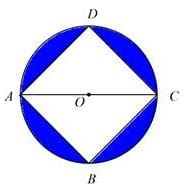Detailed Solution for RRB JE EEE (CBT I) Mock Test- 6 - Question 21 Side of the square = Perimeter/4 = 40/4 =10 cm

Diagonal AC of square = Side x √2 =10√2 cm

AC is also diameter of circle.

Radius of circle = 5√2 cm

Area of circle = 3.14 x (5√2)2 = 3.14 x 50 = 157 cm2

Area of square = 100 cm2

Area of blue portion = 157-100 = 57 cm2.

RRB JE EEE (CBT I) Mock Test- 6 - Question 22

Ramesh salary got 2 salary hikes, one of 10%, the next one of 25%. If the total increase in Ramesh salary was 24000, what is the increase in his salary from the first hike to the second Hike?

Detailed Solution for RRB JE EEE (CBT I) Mock Test- 6 - Question 22 Let Ramesh's initial salary be Rs 100x

His salary after 1st Hike = (110/100)*100x = Rs 110x

His salary after 2nd Hike = (125/100)*110x = Rs 137.5x

Given 137.5x - 100x = 24000

=> 37.5x = 24000

=> x = 640

increase in his salary from the first hike to the second Hike

= 137.5x - 110x

= 27.5x

= Rs 17,600

RRB JE EEE (CBT I) Mock Test- 6 - Question 23

Which of the following is irrational?

Detailed Solution for RRB JE EEE (CBT I) Mock Test- 6 - Question 23 Option (1)

= Product of 3rd root of 2197 and √6889

= 13 x 83

= 1079

Option (2):

= Product of 4th root of 4096 and √1377

= 8 x 9√17

= 72√17 (irrational)

Option (3):

Product of 3rd root of 3375 and √5929

= 15 x 77

= 1155

Option (4):

= Product of 4th root of 2401 and √1444

= 7 x 38

= 266

RRB JE EEE (CBT I) Mock Test- 6 - Question 24

A car wiper can clean 693 sq. inch area in its one sweep. If its length is decreased by 3.5 inch, then how much area will it clean in one sweep? (Car wiper covers a semi-circle in one sweep)

Detailed Solution for RRB JE EEE (CBT I) Mock Test- 6 - Question 24 Let original length a car wiper be L inch.

Area cleaned by the car wiper in 1 sweep

= (1/2) x (22/7) x (L)2

L2 = 693 x (7/11) = 63 x 7

L = 21 inch.

Area cleaned by car wiper in a sweep if its length decreased by 3.5 inch.

= (1/2) x (22/7) x (17.5) x (17.5)

= 481.25 sq. inch.

RRB JE EEE (CBT I) Mock Test- 6 - Question 25

A bus reached Nagpur to Amravati in 2:30 hr with the average speed of 70 km/hr. If the average speed increased by 30 km/hr. How much time it will take to cover the same distance.

Detailed Solution for RRB JE EEE (CBT I) Mock Test- 6 - Question 25

Distance = speed *time

= (70 * 150)/60 min

= 175 km

New speed = (70 + 30) km/hr = 100 km/hr

Time = (Distance )/speed

= (175 * 60)/100

= 105 min

RRB JE EEE (CBT I) Mock Test- 6 - Question 26

Two poles of equal heights are on either side of a 100 m wide road. From a point on the road, the angles of elevation of their tops are 30° and 60°. Find the height of each pole.

Detailed Solution for RRB JE EEE (CBT I) Mock Test- 6 - Question 26

Let the height of poles be h m.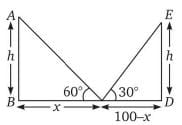Tan 60 = h/x

=> √3 = h/x

Or h = √3 x

Similarly,

1/√3 = h/(100-x)

=> 100-x = √3 h = 3x

=> x = 25

Therefore, h = 25√3 m

RRB JE EEE (CBT I) Mock Test- 6 - Question 27

The sales for the month of January was 500 units. Sales increased by 50 units every month for the next 11 months. Find the average monthly sales for the year?

Detailed Solution for RRB JE EEE (CBT I) Mock Test- 6 - Question 27 Total sales for the year = 500 + 550 + 600 + 650 +700 +750 + 800 +850 +900+ 950 + 1000 +1050 = 9300

Average monthly sales for the year = 9300/12 = 775

RRB JE EEE (CBT I) Mock Test- 6 - Question 28

Select the odd one from the following options.

a = 2.5 x 2.4 - 8 x 0.5

b = 12 x 4 x 0.5 - 16

c = 21 - 30 x 0.5

Detailed Solution for RRB JE EEE (CBT I) Mock Test- 6 - Question 28

a = 2.5 x 2.4 - 8 x 0.5 = 2

b = 12 x 4 x 0.5 - 16 = 8

c = 21 - 30 x 0.5 = 6

RRB JE EEE (CBT I) Mock Test- 6 - Question 29

A grocer bought two types of rice A and B, at Rs.20 per kg and Rs.25 per kg. If no profit and no loss occurs on selling both the items together at Rs.24 per kg, find the ratio of rice A and B.

Detailed Solution for RRB JE EEE (CBT I) Mock Test- 6 - Question 29 Let quantity of rice A and B are a and b respectively.

Total selling price = 20a + 25b

Total cost price = 24a + 24b

No profit, no loss occurred. So,

Total selling price = total cost price.

20a + 25b = 24a + 24b

4a = b

a: b = 1: 4

RRB JE EEE (CBT I) Mock Test- 6 - Question 30

In an election contested by two candidates, a candidate who got 54% of the total votes won by a margin of 288 votes. Total votes are..

Detailed Solution for RRB JE EEE (CBT I) Mock Test- 6 - Question 30 Let the total number of votes cast = x

54% of x = 46% of x + 288

8% of x = 288

x = 288 x 100/8 = 3600. Hence, the answer option is (2).

RRB JE EEE (CBT I) Mock Test- 6 - Question 31

There are two sets of figures, namely problem Figures containing five figures 1, 2, 3, 4, 5 and answer figures (a), (b), (c), (d). You have to select one Figure from the answer set, which will continue the same series as given in problem set figures.

Problem Figure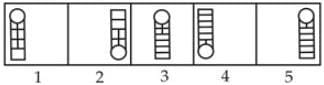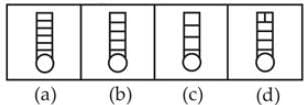Detailed Solution for RRB JE EEE (CBT I) Mock Test- 6 - Question 31 Problem figure 2 is the reverse of figure 1 with the same number of horizontal lines and vertical lines decreases by one row. Similarly, Figure 4 is revers of figure 3 and symmetrical changes in the pattern of lines. Therefore, answer figure (a) is the reverse of problem figure 5.
RRB JE EEE (CBT I) Mock Test- 6 - Question 32

DIRECTIONS: Each of the following questions consists of five figures marked A, B, C, D, and E if called the Problem figures followed by five other figures marked 1, 2, 3, and 4 called the ‘Answer figures. Select a figure from amongst the Answer Figures, which will continue the same series as established by the five Problem Figures.

Problem Figure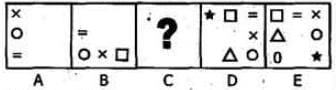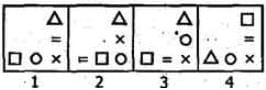Detailed Solution for RRB JE EEE (CBT I) Mock Test- 6 - Question 32 In each step, the first and the last symbols interchange positions; a new symbol appears as the first symbol (counting in a CW direction), and all the symbols move half a side of the square boundary, in the AGW direction.
RRB JE EEE (CBT I) Mock Test- 6 - Question 33

Six friends P, Q, R, S, T, and U are standing around a circular park facing towards the centre. The angle made at the centre of the circle by a straight line from P and Q is 180o, from Q and R is 120o, from R and S 180o. T is not standing on the immediate left of R while R is not on the immediate right of P. On the basis of the above information which of the following statements is definitely true?

Detailed Solution for RRB JE EEE (CBT I) Mock Test- 6 - Question 33 So, Q is standing between S and U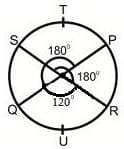RRB JE EEE (CBT I) Mock Test- 6 - Question 34

In the following diagram, circle represent police officer, triangle represent corrupt, square represents writer and rectangle represents married.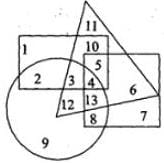The area representing unmarried police officers who are not corrupt but are writer is:

Detailed Solution for RRB JE EEE (CBT I) Mock Test- 6 - Question 34 The number representing the required area is 8.
RRB JE EEE (CBT I) Mock Test- 6 - Question 35

DIRECTIONS: In the question below are given few statements followed by some conclusion. You have to take the two given statements to be true even if they seem to be at variance with the commonly known facts and then decide which of the given conclusions logically follows from the two given statements, disregarding commonly known facts.

Statements:

All lassitude are tiredness.

Only lassitude are assiduous.

Conclusions:

Detailed Solution for RRB JE EEE (CBT I) Mock Test- 6 - Question 35 Either conclusion I or II does not follow.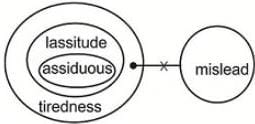RRB JE EEE (CBT I) Mock Test- 6 - Question 36

DIRECTIONS: Identify the diagram that best represents the relationship among Iron, Lead, Nitrogen.

Choose the correct venn diagram.

Detailed Solution for RRB JE EEE (CBT I) Mock Test- 6 - Question 36

There is no relation between iron, lead and nitrogen.

So,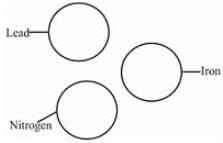RRB JE EEE (CBT I) Mock Test- 6 - Question 37

DIRECTIONS: In each of the following questions, choose the set of numbers out of the four options that is similar to the given set.

given set:(17, 35, 71)

Detailed Solution for RRB JE EEE (CBT I) Mock Test- 6 - Question 37 Double the first number + 1 = second number and double the second number + 1 = third number.
RRB JE EEE (CBT I) Mock Test- 6 - Question 38

DIRECTIONS: Study the following information to answer the given questions

In a certain code:

‘swords hidden in area’ is written as ‘white black yellow red’

‘ready swords for attack’ is written as ‘grey pink red green’

‘hidden for own safety’ is written as ‘silver grey violet white’

‘own area under attack’ is written as ‘violet blue pink black’

‘black pink yellow’ could be a code for which of the following?

Detailed Solution for RRB JE EEE (CBT I) Mock Test- 6 - Question 38

From 1 & 2

swords = red

From 1 & 3

hidden = white

From 3 & 4

own = violet

From 1 & 4

area = black

From 1

in= yellow

From 2 & 3

for= grey

From 2

From 3

safety = silver

From 4

under = blue

So, ‘black pink yellow’ is ‘Area in attack’

RRB JE EEE (CBT I) Mock Test- 6 - Question 39

Find the odd figure/word/number pair from the given responses.

Detailed Solution for RRB JE EEE (CBT I) Mock Test- 6 - Question 39 The second number is one more than the cube of the first number
RRB JE EEE (CBT I) Mock Test- 6 - Question 40

DIRECTIONS: Arrange the following words according to the English dictionary.

(a) Bhagat (b) Bhagwat (c) Bhagvati (d) Bhagirath (e) Bhagvant

Detailed Solution for RRB JE EEE (CBT I) Mock Test- 6 - Question 40 The correct arrangement is

(a) Bhagat (d) Bhagirath (e) Bhagvant (c) Bhagvati (b) Bhagwat

RRB JE EEE (CBT I) Mock Test- 6 - Question 41

DIRECTIONS: Find the odd one out from the given alternatives.

Detailed Solution for RRB JE EEE (CBT I) Mock Test- 6 - Question 41 Skip
RRB JE EEE (CBT I) Mock Test- 6 - Question 42

DIRECTIONS: Study the given information carefully and answer the given questions.

A is the mother of B. B is the sister of C. D is the son of C. E is the brother of D. F is the mother of E. G is the granddaughter of A. H has only two children — B and C.

How is F related to H?

Detailed Solution for RRB JE EEE (CBT I) Mock Test- 6 - Question 42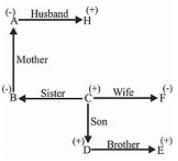F is daughter-in-law of H.

RRB JE EEE (CBT I) Mock Test- 6 - Question 43

When DRIVER = 18, ACCIDENT = 24, What is the code for ZEBRA?

Detailed Solution for RRB JE EEE (CBT I) Mock Test- 6 - Question 43 DRIVER = 6

= 6 × 3 = 18

ACCIDENT = 8

= 8 × 3 = 24

Similarly,

ZEBRA = 5

= 5 × 3 = 15

RRB JE EEE (CBT I) Mock Test- 6 - Question 44

DIRECTIONS: Find the Mirror image of the figure: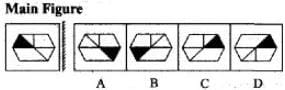Detailed Solution for RRB JE EEE (CBT I) Mock Test- 6 - Question 44 C
RRB JE EEE (CBT I) Mock Test- 6 - Question 45

DIRECTIONS: Select a correct alternative from the given alternatives to replace the question mark from the given figure.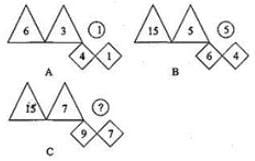Detailed Solution for RRB JE EEE (CBT I) Mock Test- 6 - Question 45 Divide the difference between the triangles by the difference between the diamonds to get the number in the circle.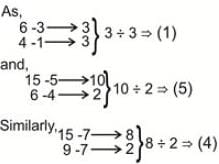RRB JE EEE (CBT I) Mock Test- 6 - Question 46

DIRECTIONS: Study the following arrangement carefully and answer the questions given below:

P 1 M R E \$ K 5 J # B 7 2 N 1 T 4 % 8 H U 9 Q J * V 6 W A 3 B D S

How many such symbols are there in the above arrangement, each of which is immediately preceded by a number and immediately followed by a consonant?

Detailed Solution for RRB JE EEE (CBT I) Mock Test- 6 - Question 46 There are no such combinations.
RRB JE EEE (CBT I) Mock Test- 6 - Question 47

Which of the words in the following options can be formed using the given word ?

CORRESPONDING

Detailed Solution for RRB JE EEE (CBT I) Mock Test- 6 - Question 47 There is no ‘T’ in the given word. So, CORRECT and REPENT cannot be formed.

There is only one ‘S’ in the given word. So, RESPONSE cannot be formed.

RRB JE EEE (CBT I) Mock Test- 6 - Question 48

DIRECTIONS: From the given responses, find the missing number in the series.

YW, US, ?, MK

Detailed Solution for RRB JE EEE (CBT I) Mock Test- 6 - Question 48 The pattern is as follows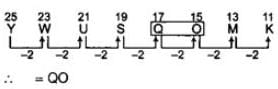RRB JE EEE (CBT I) Mock Test- 6 - Question 49

DIRECTIONS: A piece of paper is folded and cut as shown below in the questions figure. From the given answer figures, indicate how it will appear when opened?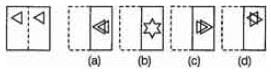RRB JE EEE (CBT I) Mock Test- 6 - Question 50

In the following question, select the related letter/word/number from the given alternatives.

Hooghly : Jute :: Ahmedabad : ?

Detailed Solution for RRB JE EEE (CBT I) Mock Test- 6 - Question 50 Hoogly is related to jute productions, similarly Ahmedabad is related to cotton type if productions.
RRB JE EEE (CBT I) Mock Test- 6 - Question 51

DIRECTIONS: An unfolded dice is given, from the answer figures which figure is similar when unfolded to the question figure.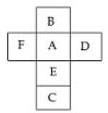Detailed Solution for RRB JE EEE (CBT I) Mock Test- 6 - Question 51 When answer figure (a) is folded it looks like the question figure.
RRB JE EEE (CBT I) Mock Test- 6 - Question 52

DIRECTIONS: In the following question, find the missing number.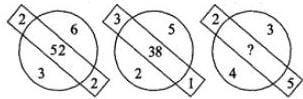Detailed Solution for RRB JE EEE (CBT I) Mock Test- 6 - Question 52 A = [(a2 + b2 + c2 + d2) — 1]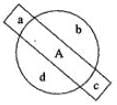So, [(22 + 42 + 32 + 52) - 1] = 54 - 1 = 53

RRB JE EEE (CBT I) Mock Test- 6 - Question 53

How many triangles are there in the given figure?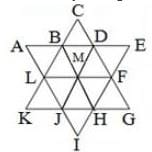Detailed Solution for RRB JE EEE (CBT I) Mock Test- 6 - Question 53

∆ABL, ∆BCD, ∆DEF, ∆FGH, ∆HIJ, ∆JKL, ∆KCG, ∆AEI, ∆LBM, ∆BMD,

∆DMF, ∆MFH, ∆HJM, ∆JLM, ∆ADJ, ∆BEH, ∆LFI, ∆CLF, ∆KBH, ∆JDG

RRB JE EEE (CBT I) Mock Test- 6 - Question 54

‘A + B’ means ‘A is the father of B’.

‘A × B’ means ‘A is the sister of B’.

‘A \$ B’ means ‘A is the wife of B’.

‘A % B’ means ‘A is the mother of B’.

‘A ÷ B’ means ‘A is the son of B’.

What should come in place of the question mark, to establish that J is the brother of T in the expression?

J ÷ P % H ? T % L

Detailed Solution for RRB JE EEE (CBT I) Mock Test- 6 - Question 54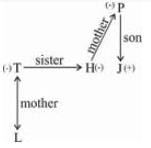RRB JE EEE (CBT I) Mock Test- 6 - Question 55

Find the missing term in the series given below.

24, 60, 120, 210, ?

Detailed Solution for RRB JE EEE (CBT I) Mock Test- 6 - Question 55 The pattern is 24 + [6 × (6 + 0)], 60 + [6×(6+4)], 120 + [6×(6+9)], ......

So, missing term= 210 + [6 × (6 + 15)] = 210 + 126 = 336.

RRB JE EEE (CBT I) Mock Test- 6 - Question 56

Who is the Prime Minister of Bhutan?

Detailed Solution for RRB JE EEE (CBT I) Mock Test- 6 - Question 56 Prime Minister of Bhutan Dr. Lotay Tshering today called on President Ram Nath Kovind at Rashtrapati Bhavan.
RRB JE EEE (CBT I) Mock Test- 6 - Question 57

Which of the following passes through India?

Detailed Solution for RRB JE EEE (CBT I) Mock Test- 6 - Question 57 Tropic of Cancer passes through India.
RRB JE EEE (CBT I) Mock Test- 6 - Question 58

Swadesh Darshan scheme was launched by Union Tourism Ministry in-

Detailed Solution for RRB JE EEE (CBT I) Mock Test- 6 - Question 58 Swadesh Darshan scheme was launched by Union Tourism Ministry in 2015.
RRB JE EEE (CBT I) Mock Test- 6 - Question 59

The moon revolve round the earth in—

Detailed Solution for RRB JE EEE (CBT I) Mock Test- 6 - Question 59 The Moon orbits Earth in the prograde direction and completes one revolution relative to the stars in approximately 27.322 days (a sidereal month).
RRB JE EEE (CBT I) Mock Test- 6 - Question 60

Which state Government has launched One District, One Product scheme recently?

Detailed Solution for RRB JE EEE (CBT I) Mock Test- 6 - Question 60 Uttar Pradesh state Government has launched One District, One Product scheme recently.
RRB JE EEE (CBT I) Mock Test- 6 - Question 61

Home ministry extends ban on eight extremist groups for how many years?

Detailed Solution for RRB JE EEE (CBT I) Mock Test- 6 - Question 61 The Ministry of Home Affairs has extended the ban on eight Meitei extremist organisations active in Manipur for five more years for their continued involvement in unlawful and violent activities.
RRB JE EEE (CBT I) Mock Test- 6 - Question 62

What is the capital of Uganda?

Detailed Solution for RRB JE EEE (CBT I) Mock Test- 6 - Question 62 Kampala is the capital of Uganda.
RRB JE EEE (CBT I) Mock Test- 6 - Question 63

Which of the following sets of Articles deals with ‘Emergency Provisions’?

Detailed Solution for RRB JE EEE (CBT I) Mock Test- 6 - Question 63 The Emergency Provisions are mentioned from Article 352 to Article 360 of the Indian Constitution. Article 352 : Proclamation of Emergency – due to external instruction or war, Article 356: Provisions in case of failure of constitutional as to financial emergency.
RRB JE EEE (CBT I) Mock Test- 6 - Question 64

Who has been appointed as the 21st Chairman of Law Commission of India?

Detailed Solution for RRB JE EEE (CBT I) Mock Test- 6 - Question 64 Justice B.S. Chauhan has been appointed as the 21st chairman of Law Commission of India in March,2018.
RRB JE EEE (CBT I) Mock Test- 6 - Question 65

The first Sultan of Delhi to issue regular currency and declare Delhi as the capital of his empire was?

Detailed Solution for RRB JE EEE (CBT I) Mock Test- 6 - Question 65 Iltutmish was the first Sultan of Delhi to issue regular currency and declare Delhi as the capital of his empire before it Badayun (1210–1214) was the capital. He introduced the silver tanka and copper jital.
RRB JE EEE (CBT I) Mock Test- 6 - Question 66

The first Indian selected For Indian Civil Service was:

Detailed Solution for RRB JE EEE (CBT I) Mock Test- 6 - Question 66 Satyendranath Tagore was the Indian to join the indian civil services.June, 1863. The ICS Act of 1861 established the Indian Civil Service. The Act of 1853 had already established the practice of recruiting covenanted civilians through competitive examinations.
RRB JE EEE (CBT I) Mock Test- 6 - Question 67

Who was the first Indian to become member of British Parliament?

Detailed Solution for RRB JE EEE (CBT I) Mock Test- 6 - Question 67 Dadabhai Naoroji.
RRB JE EEE (CBT I) Mock Test- 6 - Question 68

Who is the new Director General of Indo-Tibetan Border Police?

Detailed Solution for RRB JE EEE (CBT I) Mock Test- 6 - Question 68 The Indo-Tibetan Border Police (ITBP) is one of the five Central Armed Police Forces of India, raised on 24 October 1962, under the CRPF Act, in the wake of the Sino-Indian War of 1962. The ITBP was intended for deployment along India's border with China's Tibet Autonomous Region.
RRB JE EEE (CBT I) Mock Test- 6 - Question 69

'Mohiniyattam' is a classical dance from the Indian State of

RRB JE EEE (CBT I) Mock Test- 6 - Question 70

Because of which one of the following factors, clouds do not precipitate in deserts ?

Detailed Solution for RRB JE EEE (CBT I) Mock Test- 6 - Question 70 There are often no clouds at all in many desert regions because there is low humidity.
RRB JE EEE (CBT I) Mock Test- 6 - Question 71

Rusting of iron happens when a layer of _______ forms on the surface of iron material.

Detailed Solution for RRB JE EEE (CBT I) Mock Test- 6 - Question 71 iron + water + oxygen → hydrated iron(III) oxide ( hydrated ferric oxide)

Rust forms when oxygen reacts with iron in the presence of water/moisture.

The iron oxide reacts with oxygen to yield red rust, Fe2O3.H2O

RRB JE EEE (CBT I) Mock Test- 6 - Question 72

Which of the following is/are the characteristics of a common salt?

Detailed Solution for RRB JE EEE (CBT I) Mock Test- 6 - Question 72 Physical properties of table salt are

1. Salt is a white cubic crystals. When the salt is pure it clear. It also appear in white, grey or brownish color depending upon the purity.

2. It is odourless but has strong salty taste.

3. Solubility in water is different at different temperatures.

4. Hygroscopic(ability of a substance to attract and hold water molecules from the surroundings)

RRB JE EEE (CBT I) Mock Test- 6 - Question 73

Which of the following is/are NOT a characteristic of a solid?

Detailed Solution for RRB JE EEE (CBT I) Mock Test- 6 - Question 73 The solids are characterized by incompressibility, rigidity and mechanical strength.

The molecules, atoms or ions in solids are closely packed, i.e., they are held together by strong forces and cannot move about at random. Hence solids have definite volume, shape, slow diffusion, low vapour pressure and possess the unique property of being rigid.

RRB JE EEE (CBT I) Mock Test- 6 - Question 74

The frequency range of hearing for a normal young person is ___________ hertz.

Detailed Solution for RRB JE EEE (CBT I) Mock Test- 6 - Question 74 The frequency range of a young person is about 20 to 20,000 hertz.

The human ear is capable of hearing many of the sounds produced in nature. Any frequency that is below the human range is known as infrasound.

Bats, whales, porpoises, and dolphins use ultrasound for navigation. Most bats can detect frequencies as high as 100,000 Hz.

RRB JE EEE (CBT I) Mock Test- 6 - Question 75

Which of these is/are example(s) of Newton's 3rd Law of Motion?

Detailed Solution for RRB JE EEE (CBT I) Mock Test- 6 - Question 75 A force is a push or a pull that acts upon an object as a result of its interaction with another object.

According to Newton, whenever objects A and B interact with each other, they exert forces upon each other. These forces are called action and reaction forces and are the subject of Newton's third law of motion. Formally stated, Newton's third law is:

For every action, there is an equal and opposite reaction.

All the above given options are examples of the stated law.

RRB JE EEE (CBT I) Mock Test- 6 - Question 76

_____________ are tiny organelles inside cells that are involved in releasing energy from food hence, gaining the tag, "Power house of Cell".

Detailed Solution for RRB JE EEE (CBT I) Mock Test- 6 - Question 76 Mitochondria are tiny organelles inside cells that are involved in releasing energy from food and the process is known as cellular respiration.

It is for this reason that mitochondria are often referred to as the powerhouses of the cell. Cells that need a lot of energy, like muscle cells, can contain thousands of mitochondria.

When the breakdown products from the digestion of food find their way into the cell, a series of chemical reactions occur in the cytoplasm.

This allows some of the energy locked up in these products to be released and incorporated into the universal energy supplier in cells known as ATP (adenosine triphosphate).

ATP → ADP + P + energy for bodily function.

RRB JE EEE (CBT I) Mock Test- 6 - Question 77

Which of the following is/are a stage in the Nitrogen Cycle?

Detailed Solution for RRB JE EEE (CBT I) Mock Test- 6 - Question 77 The nitrogen cycle helps to explain as to how nitrogen flows between animals, bacteria, plants, the atmosphere, and the soil on earth.

Processes of the Nitrogen Cycle

Nitrogen fixation is the process of converting the atmospheric nitrogen (N2) into biological state nitrogen.

Nitrification is the process where the ammonium ions (NH4) are converted into nitrides, first into nitrites (NO2 - ) then into nitrate (NO3 - ) which is done by the nitrogen-fixing bacteria.

Assimilation refers to how plants and animals obtain nitrogen. Plant roots absorb nitrates from the soil into the roots then into the entire plant system.

Ammonification is also termed as the decaying process. It occurs when the plant or animal dies then decomposers such as fungi and bacteria decompose the tissues and transforms the nitrogen back into ammonium.

Denitrification is the process that changes nitrate to nitrogen gas, hence returning it into the atmosphere. This process releases the excess nitrogen in the soil back into the atmosphere

RRB JE EEE (CBT I) Mock Test- 6 - Question 78

Atomic Number of Chlorine is _____.

Detailed Solution for RRB JE EEE (CBT I) Mock Test- 6 - Question 78 Chlorine (Cl) is a member of the halogen group with an atomic number of 17.

It is the second most common halogen on Earth.

Chlorine in its pure form is yellowish-green, but its common compounds are typically colourless

Chlorine is vital for living organisms although highly concentrated pure chlorine is dangerous to living beings.

Chlorine has two stable isotopes, Cl-35 and Cl-37 while Cl-36 is radioactive.

RRB JE EEE (CBT I) Mock Test- 6 - Question 79

_____________ is the process of conversion of a solid directly into vapour.

Detailed Solution for RRB JE EEE (CBT I) Mock Test- 6 - Question 79 Sublimation is conversion of a substance from the solid to the gaseous state without its becoming liquid.

An example is the vaporization of frozen carbon dioxide (dry ice) at ordinary atmospheric pressure and temperature. The phenomenon is the result of vapour pressure and temperature relationships.

Hoarfrost is formed by direct condensation of water vapour to ice at temperatures below freezing and occurs when air is brought to its frost point by cooling.

RRB JE EEE (CBT I) Mock Test- 6 - Question 80

Which of the following are generally used as fuel for Nuclear reactors?

Detailed Solution for RRB JE EEE (CBT I) Mock Test- 6 - Question 80 Uranium is a naturally-occurring element in the Earth's crust. Traces of it occur almost everywhere, although mining takes place in locations where it is naturally concentrated.

The uranium ore requires to make fuel for nuclear. Uranium is to be extracted from the rock in which it is found, then enriched in the uranium-235 isotope, before being made into pellets that are loaded into assemblies of nuclear fuel rods.

RRB JE EEE (CBT I) Mock Test- 6 - Question 81

The boiling point of water is _______ at 1 atmospheric pressure that is, at sea level.

Detailed Solution for RRB JE EEE (CBT I) Mock Test- 6 - Question 81 The boiling point of water is 100 °C at 1 atmospheric pressure that is, at sea level.The boiling point of water depends on the atmospheric pressure, which changes according to elevation.

The boiling point of water depends on the purity of the water, atmospheric pressure. Water that contains impurities (such as salted water) boils at a higher temperature than pure water.

RRB JE EEE (CBT I) Mock Test- 6 - Question 82

Which of the following are properties of a sound wave?

Detailed Solution for RRB JE EEE (CBT I) Mock Test- 6 - Question 82 when a wave travels from one medium to another, a part of the wave undergoes reflection. The reflection of sound waves can end up with any of the two phenomena either it's an echo or reverberation.

Refraction of waves is the change in direction of waves as they pass from one medium to another.

Diffraction is the phenomena known for the bending of a wave towards the small obstacles that spreads out as a wave fronts beyond the openings.

RRB JE EEE (CBT I) Mock Test- 6 - Question 83

Pleural Membrane is associated with which organ in Human body?

Detailed Solution for RRB JE EEE (CBT I) Mock Test- 6 - Question 83 The pleural membranes enclose a fluid-filled space surrounding the lungs.

The membranes and associated fluid serve to protect the lungs and to provide lubrication. Lung tissue is delicate and easily damaged compared to muscle, bone, or connective tissue.

Protecting the lungs from damaging interactions with other tissues is important. The lungs are also constantly expanding and contracting. The pleural membranes and fluid allow the lungs to easily move within the body cavity with minimal friction from other organs.

RRB JE EEE (CBT I) Mock Test- 6 - Question 84

Which of the following is responsible for floating of the brain in the Human skull?

Detailed Solution for RRB JE EEE (CBT I) Mock Test- 6 - Question 84 In the human skull, the brain "float" in the cerebrospinal fluid. It is found within the skull and spine. This cushioning fluid is produced by the choroid plexus tissue, which is located within the brain, and flows through a series of cavities (ventricles) out of the brain and down along the spinal cord.

The cerebrospinal fluid is kept separate from the blood supply by the blood-brain barrier

RRB JE EEE (CBT I) Mock Test- 6 - Question 85

Iron, Silver are examples of ___________ of electricity whereas wood, glass are examples of __________ of electricity.

Detailed Solution for RRB JE EEE (CBT I) Mock Test- 6 - Question 85 Iron, Silver, Copper etc. are considered to be a conductor because they conduct the electron current or flow of electrons fairly easily. Most metals are good conductors of electrical current.

Insulators are materials that have the opposite effect on the flow of electrons. They do not let electrons flow very easily from one atom to another. These electrons are not free to roam around and be shared by neighbouring atoms.

Some common insulator materials are glass, plastic, rubber, air, and wood.

RRB JE EEE (CBT I) Mock Test- 6 - Question 86

________ is a softer and flexible than bone in the human body and is a connective tissue found in many areas of the body.

Detailed Solution for RRB JE EEE (CBT I) Mock Test- 6 - Question 86 Cartilage is an important structural component of the human body, a firm tissue which is softer and much more flexible than bone.

Cartilage is a connective tissue found in many areas of the body including:

• Joints between bones e.g. the elbows, knees and ankles

• Ends of the ribs

• Between the vertebrae in the spine

• Ears and nose

RRB JE EEE (CBT I) Mock Test- 6 - Question 87

Mass of an electron is ________.

Detailed Solution for RRB JE EEE (CBT I) Mock Test- 6 - Question 87 According to the Committee on Data for Science and Technology, mass of the electron, or the electron's mass is 9.109 38356 x 10-31 kg.

Mass of proton: Mass of proton is 1.6726 x 10-27 kg.

Mass of neutron: Mass of neutron is 1.6749 x 10-27 kg.

RRB JE EEE (CBT I) Mock Test- 6 - Question 88

Who is credited with discovery of X-Rays in the year 1895?

Detailed Solution for RRB JE EEE (CBT I) Mock Test- 6 - Question 88 In 1895, German physicist Wilhelm Roentgen while working in his laboratory in Würzburg, accidentally discovered the X-rays.

Roentgen was conducting experiments with a Crookes tube - basically a glass gas bulb that gives off fluorescent light when a high-voltage current is passed through it - when he noticed that the beam turned a screen 9 feet away a greenish fluorescent color, despite the tube being shielded by heavy black cardboard.

RRB JE EEE (CBT I) Mock Test- 6 - Question 89

The ash formed from burning of a Magnesium ribbon is ______ in colour.

RRB JE EEE (CBT I) Mock Test- 6 - Question 90

Chemical Formulae of Chloroform is __________

Detailed Solution for RRB JE EEE (CBT I) Mock Test- 6 - Question 90 Chloroform (IUPAC name of trichloromethane) is an organic solvent very used in chemical and pharmaceutical industries. Chloroform chemical formula is CHCl3 and its molar mass is 119.37 g mol-1

The molecule has the typical structure of a methane, which is the most related molecule due to chloroform is a methane where 3 hydrogen atoms has been substituted by 3 chloride atoms.

The molecular structure is tetrahedral.

RRB JE EEE (CBT I) Mock Test- 6 - Question 91

Near-sightedness (myopia) is a common vision condition in which you can see objects ______ to you clearly, but objects _______ are blurry.

Detailed Solution for RRB JE EEE (CBT I) Mock Test- 6 - Question 91 Near-sightedness (myopia) is a common vision condition in which you can see objects near to you clearly, but objects farther away are blurry.

Near-sightedness symptoms may include:

• Blurry vision when looking at distant objects

• The need to squint or partially close the eyelids to see clearly

• Difficulty seeing while driving a vehicle, especially at night (night myopia)

RRB JE EEE (CBT I) Mock Test- 6 - Question 92

Speed of light in vacuum is approximately ______________.

Detailed Solution for RRB JE EEE (CBT I) Mock Test- 6 - Question 92 In vacuum the speed of light is 2.997 x 108 m/s.

When light is in a vacuum, its speed has that exact value, no matter who measures it. Even if the vacuum is inside a box in a rocket traveling away from earth, both an astronaut in the rocket and a hypothetical observer on earth will measure the speed of light moving through that box to be exactly c. No one will measure a faster speed. Indeed, c is the ultimate speed limit of the universe

RRB JE EEE (CBT I) Mock Test- 6 - Question 93

____________carry blood from the tissues of the body back to the heart.

Detailed Solution for RRB JE EEE (CBT I) Mock Test- 6 - Question 93 Veins have following characteristics-

• carry blood from the tissues of the body back to the heart

• are usually positioned closer beneath the surface of the skin

• are less muscular than arteries, but contain valves to help keep blood flowing in the right direction, usually toward the heart

Haemoglobin (Hb) is a protein found in the red blood cells that carries oxygen in your body and gives blood its red colour.

RRB JE EEE (CBT I) Mock Test- 6 - Question 94

Which of the following is the strongest acid?

Detailed Solution for RRB JE EEE (CBT I) Mock Test- 6 - Question 94 Sulphuric Acid has the lowest pH compare to other acids which were mentioned in options. So, it is the strongest acid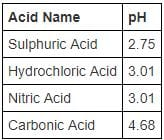RRB JE EEE (CBT I) Mock Test- 6 - Question 95

____________ is the device used for detecting the presence of small current and voltage or for measuring their magnitude.

Detailed Solution for RRB JE EEE (CBT I) Mock Test- 6 - Question 95 The galvanometer is the device used for detecting the presence of small current and voltage or for measuring their magnitude.

The galvanometer is mainly used in the bridges and potentiometer where they indicate the null deflection or zero current.

An altimeter or an altitude meter is an instrument used to measure the altitude of an object above a fixed level.

Ammeter is an instrument for measuring either direct or alternating electric current, in amperes.

A voltmeter is an electronic instrument used to measure potential between any two points in an electric or electronic circuit in volts.

RRB JE EEE (CBT I) Mock Test- 6 - Question 96

First Law of thermodynamics is equivalent to principle of ______________ of energy.

Detailed Solution for RRB JE EEE (CBT I) Mock Test- 6 - Question 96 Conservation of energy, the principle of physics according to which the energy of interacting bodies or particles in a closed system remains constant.

For example, when a pendulum swings upward, kinetic energy is converted to potential energy. When the pendulum stops briefly at the top of its swing, the kinetic energy is zero, and all the energy of the system is in potential energy. When the pendulum swings back down, the potential energy is converted back into kinetic energy. At all times, the sum of potential and kinetic energy is constant.

This version of the conservation-of-energy principle, expressed in its most general form, is the first law of thermodynamics.

RRB JE EEE (CBT I) Mock Test- 6 - Question 97

The de Broglie wavelength associated with an electron moving with a speed of 200 m/s is _______.

Detailed Solution for RRB JE EEE (CBT I) Mock Test- 6 - Question 97 The de Broglie equation is an equation used to describe the wave properties of matter, specifically, the wave nature of the electron: λ = h/mv, where λ is wavelength, h is Planck's constant (6.6x 10-34 J s), m is the mass of a particle (mass of electron= 9.1 x 10-31 kg), moving at a velocity v

Putting the values in the formulae,

λ = h/mv; λ = 6.6 x 10-34 / (9.1 x 10-31 x 200)

λ ≈ 3600 nanometre

RRB JE EEE (CBT I) Mock Test- 6 - Question 98

Which is the eye's outermost layer which is a clear, dome shaped surface covering the front of the eye?

Detailed Solution for RRB JE EEE (CBT I) Mock Test- 6 - Question 98 The cornea is the transparent part of the eye that covers the front portion of the eye.

It covers the pupil, iris, and anterior chamber.

The cornea's main function is to refract, or bend, light. The cornea is responsible for focusing most of the light that enters the eye.

The retina is a light-sensitive layer that lines the back of the eye. The retina contains photoreceptors that absorb light and then transmits those signals through the optic nerve to the brain.

RRB JE EEE (CBT I) Mock Test- 6 - Question 99

A body initially at rest moves with a constant acceleration of 15 m/s2. At what time from the initial point will it have travelled 3 kilometres?

Detailed Solution for RRB JE EEE (CBT I) Mock Test- 6 - Question 99

Using the distance-time equation for a body moving with constant acceleration, S = ut +1/2at2 where, S = distance travelled, u= initial velocity, a=acceleration, t= time taken for the body to travel distance s.

Since, the body was initially at rest, u= 0 m/s,

Hence, Putting the values in the equation, S = ut +1/2at2

3000 = 0 + ½ (15 x t2)

Or, t = 20 seconds

RRB JE EEE (CBT I) Mock Test- 6 - Question 100

___________ is a long, muscular tube that connects the mouth to stomach.

Detailed Solution for RRB JE EEE (CBT I) Mock Test- 6 - Question 100 Oesophagus is part of the digestive system, which is sometimes called the gastro-intestinal tract (GI tract). It's around 25cm (10in) long in adults.

When we swallow our food, the walls of the oesophagus squeeze together (contract) and helps to move the food down the oesophagus to the stomach.

## RRB JE Mock Test Series Electrical & Electronics Engineering

154 tests
 Use Code STAYHOME200 and get INR 200 additional OFF Use Coupon Code
Information about RRB JE EEE (CBT I) Mock Test- 6 Page
In this test you can find the Exam questions for RRB JE EEE (CBT I) Mock Test- 6 solved & explained in the simplest way possible. Besides giving Questions and answers for RRB JE EEE (CBT I) Mock Test- 6, EduRev gives you an ample number of Online tests for practice

154 tests